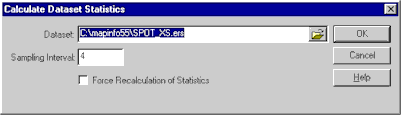Calculate Statistics

### General

This dialog allows you to calculate / recalculate the statistics stored in a dataset header.   These statistics are required by some formula to work correctly.    The Sampling Interval determines what proportion of the lines are sampled in calculating the statistics.   Checking Force Recalculating of Statistics forces the program to recalculate statistics, even if they are already present in the dataset header.

### Dialog Snapshot